When most people walk out of a casino they usually ask “Why did I lose?” This is because most casino games by nature have a negative expectation for the player. This means that for every wager that is made on a game, slot machine or table game, it gives back less than the wagered amount.

For example, when 1 million players wager \$1 each and one player wins \$500,000, the casino makes a profit of \$500,000 and the average loss per player is 50 cents. In slot machines the advertised pay back is often in the neighborhood of 97-99%.

This is over the entire life of the machine, where a machine may collect 100s of millions of dollars in action over its lifetime. Table games are slightly different because some include a skill component and the percentage advantage of the casino varies from player to player.

Understanding the math involved in casino gaming is essential in evaluating the results. The knowledge presented here is required to determine whether the results, good or bad, are statistically acceptable.

## Inside the Mathematics

Here I will introduce the mathematics of casino gaming. I will apply the analysis to the game of Blackjack. Blackjack is a game of shifting percentages. And even though the percentages are constantly changing, the cumulative percentage of the overall advantage remains constant. This is achieved by taking the sum of the advantages over all possibilities.

For example, if one hand total has an advantage of positive 5% and another hand has an advantage of -4%, then the total advantage for the two hands is +1%. When the reader understands this approach, it’s easy to translate the concepts to any other casino game with a constant advantage over the player (like slots or roulette).

## Law of Independent Trials

Most games of chance reflect the mathematical concept known as “the law of independent trials.” This states that past events have no relevance on future events. For example, when a coin is flipped there is a 50% chance that the outcome would be heads and a 50% chance that the outcome would be tails.

If the coin comes up 10 heads in a row the next flip would again have a 50% chance of coming up heads. In blackjack what happens in the past directly affects what happens in the future. Blackjack has memory, and the law of independent trials is not valid.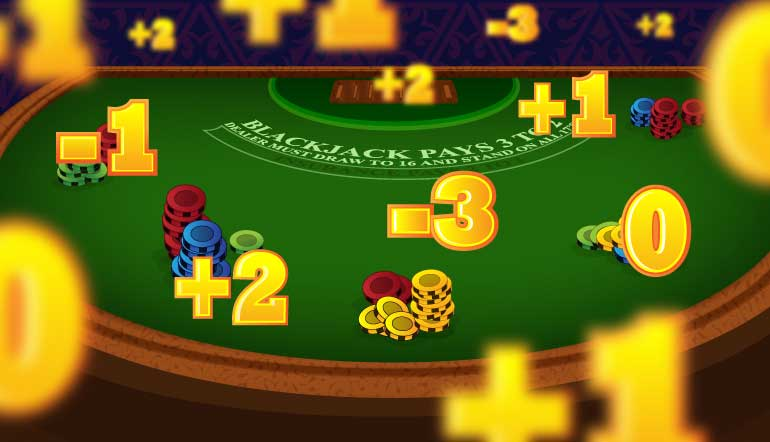In Blackjack, each card has a specific value that it adds to, or subtracts from the initial advantage that the casino has over the player. The initial advantage is derived from the rules of the game. When the cards are dealt, the advantage is either increasing or decreasing. In blackjack when an Ace or 10-value card is dealt the casino advantage over the player increases. When lower value cards are put in play (2-7) the casino advantage decreases.

To determine the amount that one expects to win or lose over a given time (either the casino or player), three key pieces of information are required. They are:

1. Bet Size
2. Number of Hands or Spins

In equation form, the statement reads:

Expected \$ win=\$bet*% advantage *# hands played

Equation 1

When we apply the expected value scenario to a flip of a coin, we know that there are two sides to a coin, so there is a 50% chance of landing on heads and a 50% chance of landing on tails. When we bet \$1 per flip, the equation for how much we expect to win over 100 flips is:

\$50=\$1 (bet)*0.5% (% advantage)*100(# hands played)

Equation 2

In this example we wagered \$100 and won \$1 on 50 of those bets. We were also able to keep the original bet of \$1 on 50 of those 100 bets. Also we lost \$1 on 50 of the bets. This leads to a zero sum game.

## Considering Variance

When a coin is flipped 100 times the outcome is rarely exactly 50 heads and 50 tails. Therefore we must introduce the concept of variance per number of events. Variance is a measure of statistical dispersion. In layman’s terms, it deals with how far away from the expected value the result of a trial or experiment might be.

Sticking with the coin flip example, variance helps answer the question of whether or not it would be surprising if we observed 45 heads out of 100 trials, or if we observed only 5 heads in 100 coin flips. The answers are no and yes. Getting only 5 heads in 100 coin flips would certainly prove you were flipping a weighted coin. Understanding this concept is crucial for evaluating casino gaming results, since proper statistical analysis determines whether or not a player or casino is being cheated.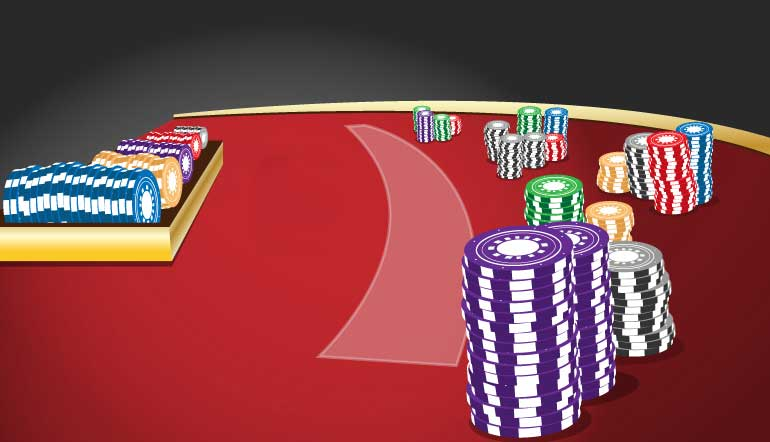Variance is usually discussed in terms of standard deviations, and that will be the case going forward in this discussion. Standard deviation is equal to the square root of the variance. The standard deviation for a series of trials is represented by the Greek letter σ (sigma) and is equal to the standard deviation of each event multiplied by the square root of the number of events. The mathematical statement reads as:

σ (total)=〖σ( event)*√(Number of Events)〗^

Equation 3

The following figure shows how likely results are to fall within one, two and three standard deviations of the expected result. In the graphical representation the expected value is indicated by the Greek letter µ and the Standard Deviation is represented by the Greek letter σ.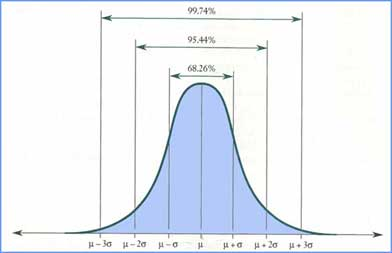According to the Gaussian distribution curve, there is just over a 68% chance that the result will be within one standard deviation, plus or minus of the expected value. There is a just over a 95% chance that the results will be within two standard deviations, plus or minus of the expected value. There is approximately a 99.9% chance that the results will be within three standard deviations at any given time.

Applying this to the scenario of 100 flips of a coin we conclude that the standard deviation for 100 trials is 10 times (square root of 100) the standard deviation for a single trial (which is 0.5), which yields a standard deviation of 5 for the 100 trial experiment.

In the coin flip scenario we expect the 50 of the 100 flips to land on heads and 50 of the 100 to land on tails. Including the standard deviation concept of plus or minus 5, there is a 68% chance that for a 100 flips of a coin the heads side will come up between 45 and 55 times. There is a 95% probability that the number of heads will be between 40 and 60 (2*σ) and a 99.9% chance that the number of heads will fall between 35 and 65 (3*σ).

## Expected Value and Standard Deviation

Applying the expected value and standard deviation equations to the betting unit of 100 dollars for a casino game with a 1% (player or house) advantage the following results are computed.

 # of Events Expected Value 1 σ 2σ 3σ 100 100 +or- 1,100 +or- 2,200 +or -3,600 10,000 10,000 +or- 11,000 +or- 22,000 +or -33,000 1,000,000 1,000,000 +or- 110,000 +or- 220,000 +or -330,000

Graphically it is represented as follows.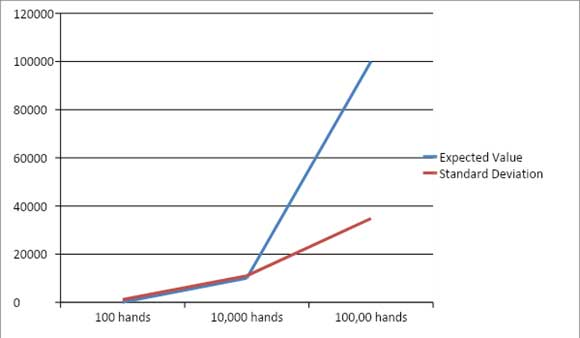As the number of events increases, the standard deviation gets smaller and smaller relative to the expected value. At some point along the curve the expected value and standard deviations intersect.

At this point there is an 84% chance that the standard deviation will be less than the expected value. This means there is an 84% chance that a profit will be made from that point forward and that your funds will never be reduced to the point where the bankroll is lower than initial starting funds. This intersection point for a 1% advantage game is shown in the following graph.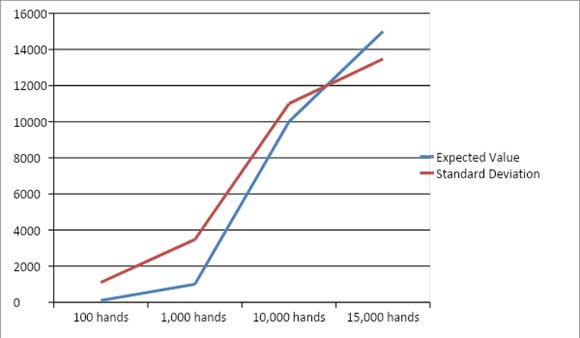FOR SIMPLICITY THE STANDARD DEVIATION VALUE IS ABSOLUTE

The intersection point between the expected value and standard deviation is just below 12,000 events. At 12,000 events there is an 84% chance that the expected value will surpass the negative standard deviation, indicating the player will not zero out their bankroll 84% of the time.

## Equivalence Point

When the overall advantage is increased the “equivalence” point, or the number of events at which the expected value equals the standard deviation, is reached in fewer events. Computing the same graph with 2% advantage the graph shows an equivalence point that is substantially lower, approximately 5000 events.FOR SIMPLICITY THE STANDARD DEVIATION VALUE IS ABSOLUTE

In the final analysis casinos are able to get to the “equivalence” point extremely fast. This makes sense because casinos are playing the game 24 hours a day, seven days a week. And because almost all players play to a disadvantage, the casinos make more and more money with less and less variance relative to their expected value.